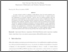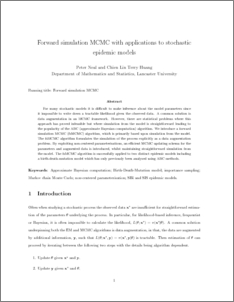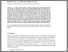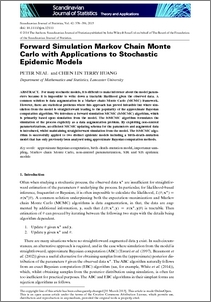Forward simulation MCMC with applications to stochastic epidemic models

Neal, Peter and Huang, Chien Lin (2015) Forward simulation MCMC with applications to stochastic epidemic models. Scandinavian Journal of Statistics, 42 (2). pp. 378-396. ISSN 0303-6898Preview
PDF (fsMCMC_sjs_final)
fsMCMC_sjs_final.pdf - Accepted VersionPreview
PDF (sjos12111)
sjos12111.pdf - Published Version

Abstract

For many stochastic models, it is difficult to make inference about the model parameters because it is impossible to write down a tractable likelihood given the observed data. A common solution is data augmentation in a Markov chain Monte Carlo (MCMC) framework. However, there are statistical problems where this approach has proved infeasible but where simulation from the model is straightforward leading to the popularity of the approximate Bayesian computation algorithm. We introduce a forward simulation MCMC (fsMCMC) algorithm, which is primarily based upon simulation from the model. The fsMCMC algorithm formulates the simulation of the process explicitly as a data augmentation problem. By exploiting non-centred parameterizations, an efficient MCMC updating schema for the parameters and augmented data is introduced, whilst maintaining straightforward simulation from the model. The fsMCMC algorithm is successfully applied to two distinct epidemic models including a birth–death–mutation model that has only previously been analysed using approximate Bayesian computation methods.

Item Type:
Journal Article
Journal or Publication Title:
Scandinavian Journal of Statistics
Uncontrolled Keywords:
/dk/atira/pure/subjectarea/asjc/1800/1804
Subjects:
Departments:
ID Code:
72712
Deposited By:
Deposited On:
28 Jan 2015 10:52
Refereed?:
Yes
Published?:
Published